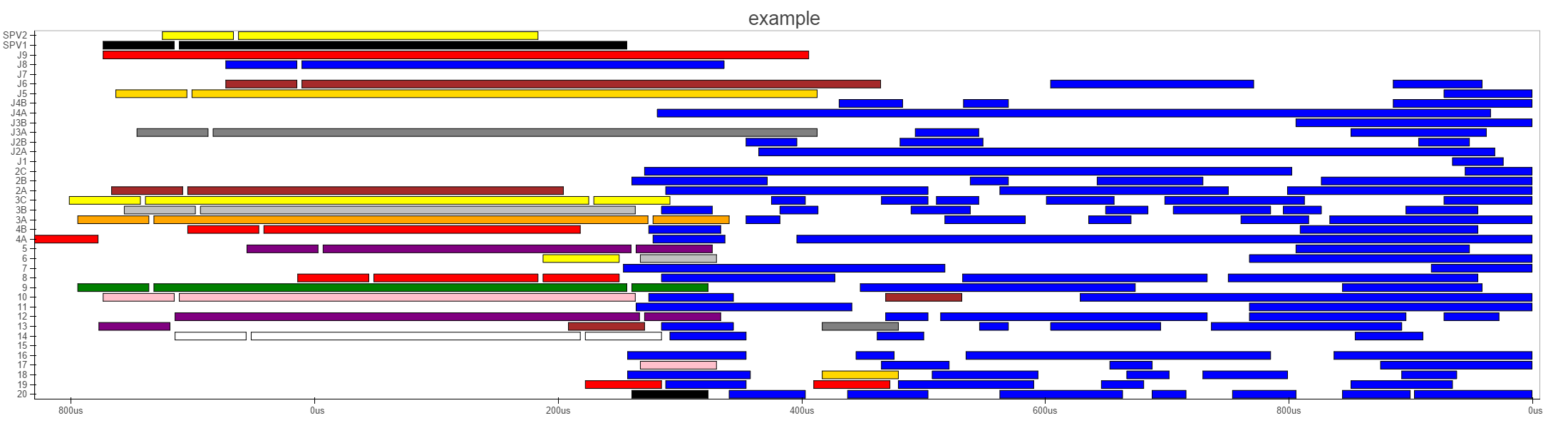# Bar chart (gant chart) problem with datetime axis

Hey all,

I am making a gant chart for a scheduling problem. I use excel number notation for the dates to be able to set the duration of the bar. When I set the x_axis_type to datetime it does convert the excel number to some type of number which does seem to represent time but I am not sure what notation it is In attachment example of the plot with the notation. I tried to fix it by using date times instead of excel times but still no result. This is the code I have now: Anyone any suggestions how to fix it?

output_file(“transferdistance.html”)

TOOLS = “resize,hover,save,pan,wheel_zoom”

p = figure(title=“example”, tools=TOOLS,x_range=[min(self.at),max(self.dt)],y_range = y_range, x_axis_type=“datetime”)

p.plot_width = 2200

p.plot_height = 600

p.toolbar_location = “left”

p.grid.grid_line_color = None

hover = p.select(dict(type=HoverTool)) source = ColumnDataSource(

data = dict(

time = [self.at + 0.5 * (self.dt-self.at) for x in flight], #exceldate representation

bay = [self.bay for x in flight],

inbound = [self.in_number for x in flight],

outbound = [self.out_number for x in flight],

duration = [self.dt-self.at for x in flight],

duration1 = [xlrd.xldate.xldate_as_datetime((self.dt - self.at+40000),book.datemode).time() for x in flight],

bayy = [self.bay+":0.15" for x in flight],

timeat = [xlrd.xldate.xldate_as_datetime(self.at,book.datemode).strftime(’%H:%M’) for x in flight],

timedt = [xlrd.xldate.xldate_as_datetime(self.dt,book.datemode).strftime(’%H:%M’) for x in flight],

timeat1 = [xlrd.xldate.xldate_as_datetime(self.at1,book.datemode).strftime(’%H:%M’) for x in flight],

timedt1 = [xlrd.xldate.xldate_as_datetime(self.dt1,book.datemode).strftime(’%H:%M’) for x in flight],

timeat2 = [xlrd.xldate.xldate_as_datetime(self.at2,book.datemode).strftime(’%H:%M’) for x in flight],

timedt2 = [xlrd.xldate.xldate_as_datetime(self.dt2,book.datemode).strftime(’%H:%M’) for x in flight],

timeat3 = [xlrd.xldate.xldate_as_datetime(self.at3,book.datemode).strftime(’%H:%M’) for x in flight],

timedt3 = [xlrd.xldate.xldate_as_datetime(self.dt3,book.datemode).strftime(’%H:%M’) for x in flight],

color = [self.color for x in flight],

connection =[self.connection for x in flight],

origin = [self.org for x in flight],

dest = [self.dest for x in flight]

)

)

hover.tooltips=OrderedDict([

(“inbound”, “@inbound”),

(“outbound”, “@outbound”),

(“ac_type”, “@ac_type”),

(“at”, “@timeat”),

(“dt”, “@timedt”),

(“at1”, “@timeat1”),

(“dt1”, “@timedt1”),

(“at2”, “@timeat2”),

(“dt2”, “@timedt2”),

(“at3”, “@timeat3”),

(“dt3”, “@timedt3”),

(“connection”, “@connection”),

(“dest”, “@dest”),

(“origin”, “@origin”)

])

p.rect(“time”,“bay”,“duration”,0.8,line_color=“black”,color=“color”,source=source)

show§